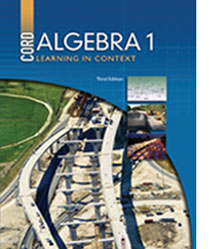# Algebra 1 - 3rd Edition

## Chapter 2: Measurement

Some links are repeated for use with more than one lesson.

### This site applies to the entire chapter.

http://www.factmonster.com/ipka/A0876863.html
This page contains lists of all basic forms of measure along with conversion rates between them.

### 2.1 Metric Measurements

http://www.mathsisfun.com/measure/metric-system.html
This site contains the basic metric measurements with real-life examples of things of each size.

http://www.factmonster.com/ipka/A0769580.html

### 2.2 Rates and Unit Analysis

This site shows how to convert units of measurement and shares examples.

### 2.3 Scale Drawings

http://www.virtualnerd.com/pre-algebra/ratios-proportions/scale-models/
This page contains links to brief video explanations of scale drawings and how to use ratios and proportions to find dimensions on such drawings.

### 2.4 Perimeter and Area of Polygons and Circles

http://www.regentsprep.org/regents/math/algebra/as1/pracarea.htm
This site contains lessons on the area of polygons and circles with practice examples.

### 2.5 Surface Area and Volume

This site contains formulas for surface area and volume of cylinders, cones, pyramids, spheres, and prisms.

http://www.learner.org/interactives/geometry/area.html
This site contains real life examples of surface area and volume of rectangles and cylinders along with explanations of how to find them.

### 2.6 Precision and Accuracy

http://www.mathsisfun.com/accuracy-precision.html
This page explains precision and accuracy and has diagrams to show the differences between them.

http://www.carlton.srsd119.ca/chemical/Sigfigs/accuracy_and_precision.htm
This article defines precision and accuracy and explains how they would change measurements.

### 2.7 Error Analysis

http://elsmar.com/pdf_files/Measurement_Error_Analysis.doc
This page explains measurement error analysis while defining terms associated with error analysis